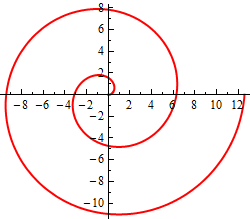Paul's Online Notes
Home / Calculus II / Parametric Equations and Polar Coordinates / Arc Length with Polar Coordinates
Show Mobile Notice Show All Notes Hide All Notes
Mobile Notice
You appear to be on a device with a "narrow" screen width (i.e. you are probably on a mobile phone). Due to the nature of the mathematics on this site it is best views in landscape mode. If your device is not in landscape mode many of the equations will run off the side of your device (should be able to scroll to see them) and some of the menu items will be cut off due to the narrow screen width.

### Section 9.9 : Arc Length with Polar Coordinates

We now need to move into the Calculus II applications of integrals and how we do them in terms of polar coordinates. In this section we’ll look at the arc length of the curve given by,

$r = f\left( \theta \right)\hspace{0.5in}\alpha \le \theta \le \beta$

where we also assume that the curve is traced out exactly once. Just as we did with the tangent lines in polar coordinates we’ll first write the curve in terms of a set of parametric equations,

\begin{align*}x & = r\cos \theta & \hspace{0.75in} y & = r\sin \theta \\ & = f\left( \theta \right)\cos \theta & \hspace{0.75in} & = f\left( \theta \right)\sin \theta \end{align*}

and we can now use the parametric formula for finding the arc length.

We’ll need the following derivatives for these computations.

\begin{align*}\frac{{dx}}{{d\theta }} & = f'\left( \theta \right)\cos \theta - f\left( \theta \right)\sin \theta & \hspace{0.75in}\frac{{dy}}{{d\theta }} & = f'\left( \theta \right)\sin \theta + f\left( \theta \right)\cos \theta \\ & = \frac{{dr}}{{d\theta }}\cos \theta - r\sin \theta & \hspace{0.75in}& = \frac{{dr}}{{d\theta }}\sin \theta + r\cos \theta \end{align*}

We’ll need the following for our $$ds$$.

\begin{align*}{\left( {\frac{{dx}}{{d\theta }}} \right)^2} + {\left( {\frac{{dy}}{{d\theta }}} \right)^2} & = {\left( {\frac{{dr}}{{d\theta }}\cos \theta - r\sin \theta } \right)^2} + {\left( {\frac{{dr}}{{d\theta }}\sin \theta + r\cos \theta } \right)^2}\\ & = {\left( {\frac{{dr}}{{d\theta }}} \right)^2}{\cos ^2}\theta - 2r\frac{{dr}}{{d\theta }}\cos \theta \sin \theta + {r^2}{\sin ^2}\theta \\ & \hspace{0.75in} + {\left( {\frac{{dr}}{{d\theta }}} \right)^2}{\sin ^2}\theta + 2r\frac{{dr}}{{d\theta }}\cos \theta \sin \theta + {r^2}{\cos ^2}\theta \\ & = {\left( {\frac{{dr}}{{d\theta }}} \right)^2}\left( {{{\cos }^2}\theta + {{\sin }^2}\theta } \right) + {r^2}\left( {{{\cos }^2}\theta + {{\sin }^2}\theta } \right)\\ & = {r^2} + {\left( {\frac{{dr}}{{d\theta }}} \right)^2}\end{align*}

The arc length formula for polar coordinates is then,

$L = \int{{ds}}$

where,

$ds = \sqrt {{r^2} + {{\left( {\frac{{dr}}{{d\theta }}} \right)}^2}} \,d\theta$

Let’s work a quick example of this.

Example 1 Determine the length of $$r = \theta$$ $$0 \le \theta \le 1$$.
Show Solution

Okay, let’s just jump straight into the formula since this is a fairly simple function.

$L = \int_{{\,0}}^{{\,1}}{{\sqrt {{\theta ^2} + 1} \,d\theta }}$

We’ll need to use a trig substitution here.

$\theta = \tan x\hspace{0.5in}d\theta = {\sec ^2}x\,dx$ \begin{align*}\theta & = 0\hspace{0.5in}0 = \tan x\hspace{0.5in}x = 0\\ \theta & = 1\hspace{0.5in}1 = \tan x\hspace{0.5in}x = \frac{\pi }{4}\end{align*} $\sqrt {{\theta ^2} + 1} = \sqrt {{{\tan }^2}x + 1} = \sqrt {{{\sec }^2}x} = \left| {\sec x} \right| = \sec x$

The arc length is then,

\begin{align*}L &= \int_{{\,0}}^{{\,1}}{{\sqrt {{\theta ^2} + 1} \,d\theta }}\\ & = \int_{{\,0}}^{{\,\frac{\pi }{4}}}{{{{\sec }^3}x\,dx}}\\ & = \left. {\frac{1}{2}\left( {\sec x\tan x + \ln \left| {\sec x + \tan x} \right|} \right)} \right|_0^{\frac{\pi }{4}}\\ & = \frac{1}{2}\left( {\sqrt 2 + \ln \left( {1 + \sqrt 2 } \right)} \right)\end{align*}

Just as an aside before we leave this chapter. The polar equation $$r = \theta$$ is the equation of a spiral. Here is a quick sketch of $$r = \theta$$ for $$0 \le \theta \le 4\pi$$.